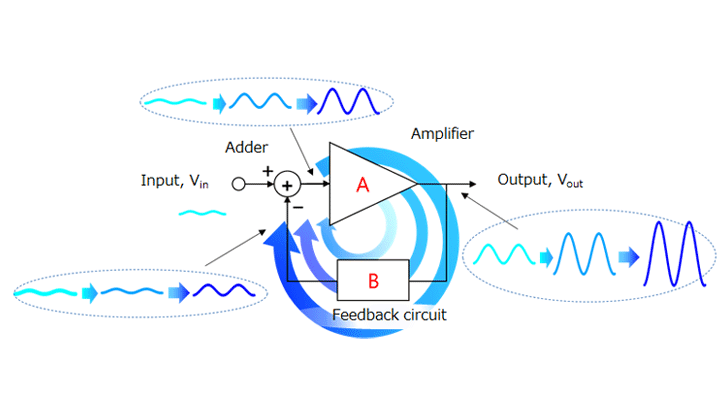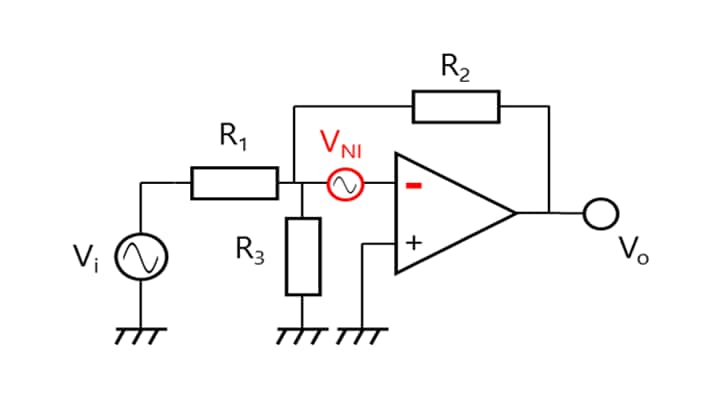# 3.4. Noise gain and signal gain

The previous section described different types of noise generated by the op-amp. As discussed, the internal noise of an op-amp is defined as equivalent input noise voltage. Figure 3-12 shows a simplified equivalent circuit. The op-amp represented by the triangle is the ideal one. Since Figure 3-12 expresses the equivalent input noise voltage (VNI) as a difference in voltage between VIN(+) and VIN(-), it is not significant whether VNI is inserted in series with VIN(+) or VIN(-).

Next, let’s consider noninverting and inverting amplifiers using this model.
Figure 3-13 shows an inverting amplifier, and Figure 3-14 shows a noninverting amplifier. Both the inverting and noninverting amplifiers have an equivalent input noise source (VNI) inserted in series with the Vin(-) input of the ideal op-amp. These amplifiers have a signal gain of AV as discussed in Sections 2.4 and 2.5.

Using the principle of superposition, signal and noise sources can be considered separately. Let’s calculate the gain for the noise source. At this time, Vi is regarded as being short-circuited according to the principle of superposition.
Since the VIN(+) input is grounded in Figure 3-13, the VIN(-) input can also be regarded as being grounded. Therefore, the potential at the intersection of R1 and R2 becomes VNI.
Since the current flowing through R1 (I1) does not flow to the op-amp, all of I1 flows through R2.

I1 = VNI / R1

Hence, the noise voltage at VO (VNO) is calculated as:

VNO = VNI + R2 × VNI / R1 = VNI × (1 + R2 / R1)

Since the noise gain (AN) is equal to VNO/VNI,

AN = 1 + R2 / R1

In this way, the gain of the noise generated in an op-amp might be different from that of the signal gain. This gain is called a noise gain.

This concept of noise gain can be used as follows:

• Converting the equivalent input noise into output noise
• Calculating the effect of the input offset voltage on the output
• Calculating the oscillation margin

As described above, the concept of noise gain is important for circuits using an op-amp.Figure 3-15 Growth of an unwanted signal through a feedback circuit

Next, the following paragraphs briefly describe the oscillation margin.
Except for oscillators, oscillation means an unwanted fluctuation of a signal at an unintended frequency. A source of oscillation such as unwanted noise circulates through a feedback loop, developing into oscillation, as described in Section 2.3.Figure 3-16 Example of a circuit to increase an oscillation margin

The source of oscillation is random noise. It is applied as a difference in voltage between the VIN(+) and VIN(-) inputs of an op-amp. In other words, it is the equivalent input noise voltage (VNI) discussed above.
It is important to determine the oscillation immunity based on the noise gain. As described above, the noise gain of typical inverting and noninverting amplifiers can be calculated using the signal gain equation for noninverting amplifiers.
The concept of the noise gain can be used to provide a margin for oscillation (i.e., increase the noise gain).

Figure 3-16 shows an example of increasing the oscillation margin without changing the signal gain with an inverting amplifier.
Let’s consider Vi and VNI separately using the principle of superposition.
(VNI is regarded as being short-circuited when considering Vi whereas Vi is regarded as being short-circuited when considering VNI.)

From the concept of a virtual short, both the VIN(-) and VIN(+) inputs are regarded as being grounded.
Therefore, since the voltage across R3 is equal to the GND potential at a signal gain of AV (= Vo/Vi), no current flows through R3. Hence, AV = -R2/R1, which is identical to the equation for a basic inverting amplifier.
Since Vi is short-circuited at a noise gain of AN (= Vo/VNI), Vi = R1 // R3. Hence, AN = 1 + R2 / (R1 // R3), which is higher than the noise gain for basic inverting amplifiers, AN = 1 + R2 / R1. This means that this circuit provides a larger oscillation margin than a basic inverting amplifier.
However, since the concept of the noise gain is exactly the same as that of the input offset voltage, the oscillation margin increases at the expense of an increase in input offset voltage.

## Chapter3 Electrical characteristics

3.2. Common-mode input voltage range (CMVIN) and common-mode input signal rejection ratio (CMRR)

## Related information

• Products
• Application Notes
• FAQs
• Parametric Search
• Stock Check & Purchase
A new window will open